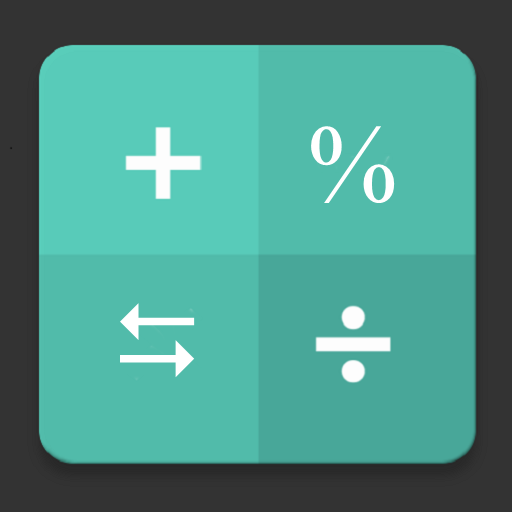# Smart Calculator - All in one

##### About Smart Calculator - All in one
The everyday use all in one lightweight calculator with multiple features & easy-to-use interface. A multi-functional calculator & converter designed to perform simple to complex calculations at your finger tips.

Collection of smart tools for INSTANT SOLUTION to your everyday problems.

Calculators
Basic & Scientific calculator
GST/Sales Tax/VAT calculator
Loan calculator: EMI, Compare Loan, Loan Affordability, Loan Amortization
Investment calculator: FD, RD, ROI, SIP, CAGR
Percentage calculator, Markup & Margin, Profit & Loss
Unit Price calculator : Find cheapest price
Health Calculator: BMI, BMR, Body Fat % & Daily Calories burn, Ideal Weight
Date Calculator: Age, Duration, ± Date, Days Counter, Time-sheet, Working Days
Discount calculator
Tip calculator
Math solver

Converters
Unit converter
Currency converter

Key Features

General & Scientific Calculator
- Facilitates instant & accurate calculations from simple to complex
- Store value for later using memory keys
- Track your calculations with History. Write a short note for important calculations
- Undo result, quick copy & share
- Number to Word conversion
- Extra functions on long-press

Unit Converter
- Simple, smart & elegant tool with more than 200 unit converters that are used in daily life
- Supported conversion categories:- Length & Distance, Weight, Area, Volume, Temperature, Cooking, Number Base, Digital Storage, Number Conversion, Data Transfer, Time, Speed, Force, Power, Fuel, Energy, Angle, Density, Acceleration, Frequency, Torque.

Currency converter
- Foreign exchange rates of 170 countries currency
- Available offline

GST /Sales Tax/VAT Calculator
-Include(+) or Exclude(-) Tax from given amount with single tap.
- 5 Pre-provided tax rates can be customized as per your country rates.

Interest Calculator
-Calculate interest & maturity amount on your saving through Simple Interest, Compound Interest, RD, SIP & Doubling Time.
-Check your investment opportunities through ROI

Percentage Calculator
-Simple percentage, % increase/decrease, % change, what %, fraction to %
-Calculate Markup percentage, Margin percentage & total profit based on cost & selling price

Health Calculator
- BMI, BMR, Body Fat %, Daily Water Intake based on your age, gender, height & weight. Calculate daily calorie requirement as per selected activity level.

Date Calculator
-Age calculator & Birthday tracking
-Duration between two dates/times
-Add/Subtract duration to date & calculate final date & time.
-Days/Hours Countdown. Never miss your important events

Equation Solver
- Linear equations with one,two & three variables

Loan Calculator
-Calculate your EMI, total interest & final amount with amortization chart in a easiest way.
-Compare two loans to know which one is better for you.
-Calculate how much loan you can afford based on your max monthly EMI.

Discount Calculator
-Calculate or confirm discounts quickly on original price.
-Find out how much you save for an item.

Tip Calculator
-Calculate the tip percentage or tip amount & split the bill among number of persons on table.
-Save time when you use the bill splitter, and tip exactly what you mean to, no more, no less.

Unit Price Calculator
-Compare prices of two or more products having different quantities of same unit & buy cheapest price item.

Number to word on click of every result field

Customization
- Calculator is fully customized where user can set no of decimal places, date format, number format, default calculator, choose from attractive & user friendly themes etc.

- More features will be added. Stay in touch!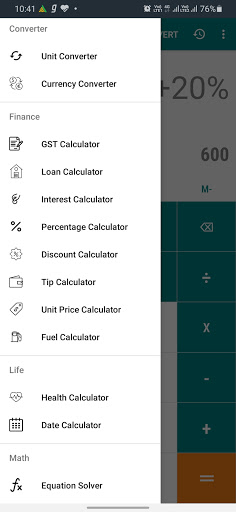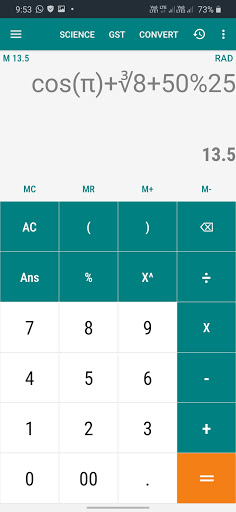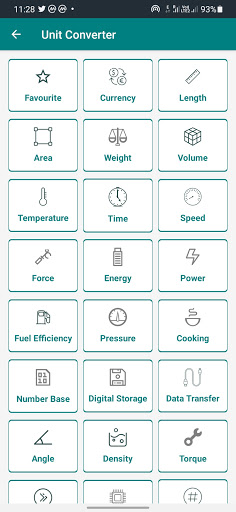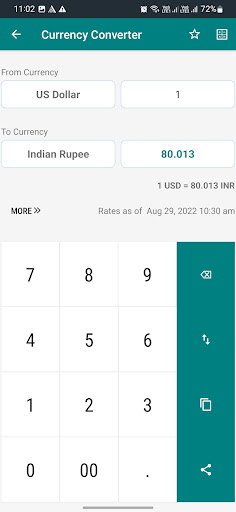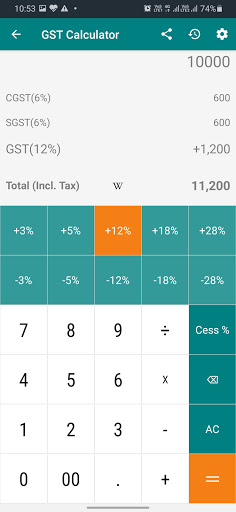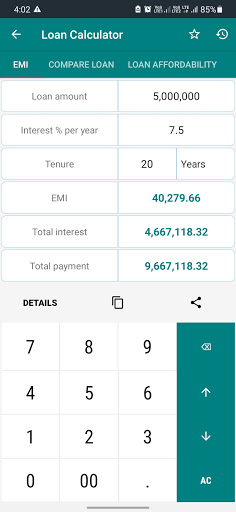• Bug fixes